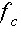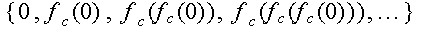THE MANDELBROT SET

The Mandelbrot set M arises from the family of quadratic functions, where z is a complex input and c is a complex parameter. One way to define this set is to fix a value of c=c1+i*c2 and compute the orbit of z=0 underas follows:.

If this set of complex numbers is bounded in the complex plane, then c belongs to M, and vice versa. The significance of  z=0 is that it is the critical value of.
A second, equivalent, way to define the Mandelbrot set is given in terms of filled Julia sets. If one fixes a value of c=c1+i*c2, the filled Julia set Kc is the set of complex inputs z for which the orbitis bounded in the complex plane. Note that while the Mandelbrot set M is a set in the c-plane, each filled Julia set Kc, which depends upons the value of c,  is a set in the z-plane. It ends up that the Mandelbrot set M consists precisely of those values of for which Kc is connected.

The Java applet below draws in black the Mandelbrot set on the viewing window [xmin, xmax] x [ymin, ymax].
The coarseness level, an integer between 1 and 9 inclusive, determines the quality of the image. A coarseness level of 9 produces the highest quality image but takes longer to produce.
• To change the viewing window and/or the coarseness level, edit the desired fields and push the "redraw" button.
• To zoom in at a point on the graph, click the mouse at the desired point, and then hit the "zoom" button. (The zoom factor is 4 in both the horizontal and vertical directions.)
• To view the filled Julia set Kc associated with a particular value of c = (c1,c2), click the mouse at the desired value of c, and then hit the "julia" button.
• The "reset" button draws the Mandelbrot set using the default coarseness level (7) and the default viewing window ([-2, .5] x [-1.25, 1.25]).
• The "redraw" button redraws the Mandelbrot set using the current settings.
• By changing alpha and beta, you may view Mandelbrot sets associated with other binary number systems number systems.
A tip for zooming: The applet uses a standard escape time algorithm to determine whether each point (c1,c2) belongs in the Mandelbrot set. For points belonging to the Mandelbrot set, this algorithm takes longer than it does for points not in the set.  Consequently, when you zoom, the more points in black (i.e. in the Mandelbrot set) that fall within your new window, the longer the time needed to create the new image.